Download Presentation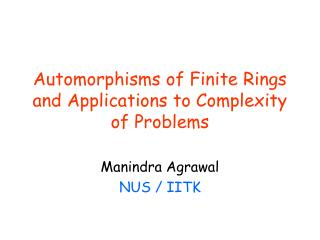Automorphisms of Finite Rings and Applications to Complexity of Problems

# Automorphisms of Finite Rings and Applications to Complexity of Problems - PowerPoint PPT Presentation

Automorphisms of Finite Rings and Applications to Complexity of Problems. Manindra Agrawal NUS / IITK. Motivation. Automorphisms of an algebraic structure capture its symmetries. Many properties can be proved by analyzing the automorphism group of the structure. Examples in Mathematics.I am the owner, or an agent authorized to act on behalf of the owner, of the copyrighted work described.
Download Presentation## Automorphisms of Finite Rings and Applications to Complexity of Problems

An Image/Link below is provided (as is) to download presentation

Download Policy: Content on the Website is provided to you AS IS for your information and personal use and may not be sold / licensed / shared on other websites without getting consent from its author.While downloading, if for some reason you are not able to download a presentation, the publisher may have deleted the file from their server.

- - - - - - - - - - - - - - - - - - - - - - - - - - E N D - - - - - - - - - - - - - - - - - - - - - - - - - -
Presentation Transcript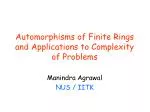### Automorphisms of Finite Rings and Applications to Complexity of Problems

Manindra Agrawal

NUS / IITK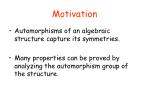Motivation
• Automorphisms of an algebraic structure capture its symmetries.
• Many properties can be proved by analyzing the automorphism group of the structure.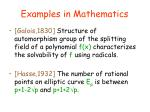Examples in Mathematics
• [Galois,1830] Structure of automorphism group of the splitting field of a polynomial f(x) characterizes the solvability of f using radicals.
• [Hasse,1932] The number of rational points on elliptic curve Ep is between p+1-2p and p+1+2p.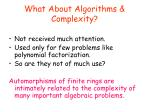What About Algorithms & Complexity?
• Not received much attention.
• Used only for few problems like polynomial factorization.
• So are they not of much use?

Automorphisms of finite rings are intimately related to the complexity of many important algebraic problems.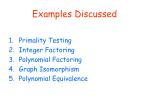Examples Discussed
• Primality Testing
• Integer Factoring
• Polynomial Factoring
• Graph Isomorphism
• Polynomial Equivalence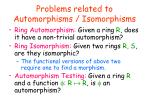Problems related to Automorphisms / Isomorphisms
• Ring Automorphism: Given a ring R, does it have a non-trivial automorphism?
• Ring Isomorphism: Given two rings R, S, are they isomorphic?
• The functional versions of above two require one to find a morphism.
• Automorphism Testing: Given a ring R and a function : R  R, is  an automorphism?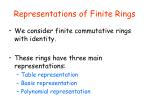Representations of Finite Rings
• We consider finite commutative rings with identity.
• These rings have three main representations:
• Table representation
• Basis representation
• Polynomial representation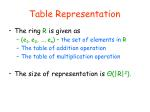Table Representation
• The ring R is given as
• (e1, e2, …, en) – the set of elements in R
• The table of addition operation
• The table of multiplication operation
• The size of representation is Θ(|R|2).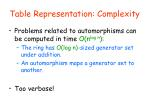Table Representation: Complexity
• Problems related to automorphisms can be computed in time O(nlog n):
• The ring has O(log n)-sized generator set under addition.
• An automorphism maps a generator set to another.
• Too verbose!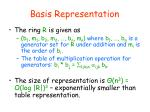Basis Representation
• The ring R is given as
• (b1, m1, b2, m2, …, bn, mn) where b1, …, bn is a generator set for R under addition and miis the order of bi.
• The table of multiplication operation for generators: bi * bj = 1≤k≤nijk bk.
• The size of representation is Θ(n3) = O(log |R|)3– exponentially smaller than table representation.Basis Representation: Complexity
• Problems related to automorphisms are in the class FPAM coAM[Kayal-Saxena,2004]:
• An automorphism/isomorphism is a linear map on additive generator set.
• So guess-and-verify technique works.
• A variant of Graph Isomorphism in coAM proof works.Polynomial Representation
• The ring R is given as
• Zm[X1, …, Xn] / (f1, …, fk) where X1, …, Xn is a generator set for R under addition and multiplication and (f1, …, fk) is the ideal of polynomials satisfied by X1, …, Xn.
• Each fi is given as an arithmetic circuit.
• The size of representation can be exponentially smaller than basis representation:
• Example: F2[X1, …, Xn] / (X12, …, Xn2)Polynomial Representation: Complexity
• Problems related to automorphisms are NP-hard:
• An automorphism is completely specified by its action on X1, …, Xn.
• Verifying membership in the ideal (f1, …, fk) can be hard (EXPSPACE-complete in general).
• Ring Automorphism problem is NP-hard.
• Ring Isomorphism problem is coNP-hard.
• Too compact!So the best representation, from the complexity perspective, is basis representation.
• Often, basis and polynomial representations have similar sizes.
• In such cases, we use polynomial representation as it is most natural one.Automorphism Testing  Primality Testing

Fermat’s Little Theorem: If n is prime then the map (x) = xn (mod n) is the trivial automorphism of ring Zn.

• Converse is not true.
• Even if it were, it is expensive to test that the map is indeed an automorphism.
• These problems can be eliminated!Automorphism Testing  Primality Testing
• Let R = Zn[Y] / (Yr – 1) for some r > 0 and define : R  R as (x) = xn.

Observation:  is an automorphism of R iff for every g(Y)  R,

gn(Y) = (g(Y)) = g((Y)) = g(Yn).Automorphism Testing  Primality Testing

[A-Kayal-Saxena,2002]: For suitably chosen “small” r, if (Y + a)n = Yn + a in R for 1 ≤ a ≤ √r log n, then either n is prime or has a divisor < r.

• Above is a slight generalization of the original statement.Automorphism Testing  Primality Testing
• Let ring S = Zn[Y] / (Y2r – Yr).
• The AKS theorem translates to:

Theorem: (1) n is prime iff  is an automorphism in S.

(2) is an automorphism in S iff (Y + a) = (Y) + a for 1≤ a≤ √r log n.Automorphism Testing  Polynomial Factoring
• Let f be a polynomial of degree d in Fq[Y].
• Let R = Fq[Y] / (f) and (x) = xq.

Observation: (1)  is an automorphism in R and d is the trivial automorphism.

(2)k is trivial iff degrees of all irreducible factors of f divide k.

(3)k is trivial iff Yqk = k(Y) = Y.Automorphism Testing  Polynomial Factoring
• This allows to test for irreducibility of f as well as separate distinct degree factors of f:
• Fork = 1toddo: computegcd(f, Yqk – Y).Automorphism Testing  Polynomial Factoring
• Finding equal degree factors of f can be reduced to finding roots of a related polynomial in Fq:
• Find at(Y)  R \ Fq, with(t(Y)) = t(Y).[use linear algebra]
• Letg(x) = Res( t(Y) – x, f(Y) ).
• For a rootαofg, gcd( t(Y) – α, f(Y) )is non-trivial.Automorphism Testing  Polynomial Factoring
• Roots of g can be computed using distinct degree factorization method.
• Works in randomized polynomial time.Finding Ring Automorphism  Integer Factoring
• Quadratic Sieve, Number Field Sieve: the fastest two known method for factoring integers.
• Both aim to find a and b in Zn, a ≠ ± b, a2 = b2 (mod n).
• Given such a and b, gcd(a+b, n) is non-trivial.

These methods are equivalent to finding an automorphism in a special ring.Finding Ring Automorphism  Integer Factoring
• Let R = Zn[Y] / (Y2 – 1) for odd n.

Observation: x  x and x  –x are two straightforward automorphisms in R.

Lemma: Let  be any automorphism of R. Then, (Y) = cY with c2 = 1 (mod n).Finding Ring Automorphism  Integer Factoring

Proof: Let (Y) = cY + d. Then,

0 = (Y2 – 1) = (cY+d)2 – 1

= 2cdY + c2 + d2 – 1.

Since  is an automorphism, (c, n) = 1. Thus, d = 0 and c2 = 1 in Zn. □

So for any third automorphism, c ≠ ± 1.Therefore, finding a third automorphism is equivalent to factoringn.Finding Ring Automorphism  Integer Factoring
• Conversely, finding ring automorphism can be reduced to integer factoring.
• [Kayal-Saxena,2004] showed how:
• Given ring R, split it as a sum of localrings using integer and polynomial factoring oracles.
• For each local ring, it is easy to find a non-trivial automorphism if it exists.Finding Ring Automorphism  Integer Factoring
• There are many other connections too.
• [Kayal-Saxena,2004] showed that integer factoring reduces to:
• Counting number of automorphisms of Zn[Y] / (Y2).
• Finding any non-trivial automorphism of Zn[Y] / (f),f a random degree 3 poly.
• Finding any isomorphism between Zn[Y] / (Y2-1) and Zn[Y] / (Y2-a2),a randomly chosen from Zn.Ring Isomorphism  Graph Isomorphism
• Shown in [Kayal-Saxena,2004].
• Here, we give a different, more general proof.
• Let G = (V, E) be a graph on n vertices.
• Define polynomial pG as:

pG(x1,…,xn) = (i,j)E xi xj.

• Define polynomial ideal IG as:

IG(x1,…,xn) = (pG(x1,…,xn), {xi2}1 ≤i ≤ n,

{xixjxk}1≤ i < j < k ≤ n).Ring Isomorphism  Graph Isomorphism
• Let Rq,G = Fq[Y1,…,Yn] / IG(Y1,…,Yn).

Theorem: Graphs G1 and G2 are isomorphic iff either G1 = G2 = Km Dn-m or rings Rq,G1 and Rq,G2 are isomorphic.

Here, Dn-m is a collection of n-m isolated vertices and q any odd prime power.Ring Isomorphism  Graph Isomorphism

Proof: If the graphs are isomorphic via , the rings are isomorphic via (Yi) = Y(i).

Suppose the rings are isomorphic and G2≠ Km Dn-m for any m.

Let  be an isomorphism,

(Yi) = ai + 1≤ j≤ n bijYj + 1≤ j < k≤ n cijk YjYkRing Isomorphism  Graph Isomorphism

Since (Yi)2 = (Yi2) = 0:

0 = (Yi)2 = ai2+higher degree terms,

implying that ai = 0.

So:

0 = (Yi)2 = 2 1≤ j < k≤ n bijbik YjYk.Ring Isomorphism  Graph Isomorphism

If two or morebi’s are non-zero, pG2 must divide (Y)2.

This implies G2 =Km Dn-m. Not possible.

If allbi’s are zero then (YiYt) = 0. Not possible.

So, exactly one of bi’s is non-zero.Ring Isomorphism  Graph Isomorphism

Let (i) = j where bij is non-zero.

If (i) = (t), then (YiYt) = 0. Not possible.

So  is a permutation on [1,n].Ring Isomorphism  Graph Isomorphism

Also:

0 = (pG1) = (i,j)E1(Yi)(Yj)

= (i,j)E1 bi,(i)bj,(j) YiYj.

So pG2 must divide above.

This means (pG1) is a constant multiple of pG2 implying that  is an isomorphism.Polynomial Equivalence

The Problem: Given two polynomialsfandginF[x1,…,xn],test if there exists an invertible linear transformationTsuch that

g(x1,…,xn) = f(Tx1,…,Txn).

• [Thierauf,1998] proved it is in NP  coAM when T is required to be a permutation.
• His proof works for arbitrary linear transformations too.Polynomial Equivalence
• Polynomial equivalence for d-forms (homogeneous polynomials of degree d) is well-studied.
• Witt’s theorem implies a polynomial time algorithm for quadraticforms.
• No such algorithm is known for cubicforms.
• There is even a cryptosystem based on (presumed) difficulty of deciding equivalence between collections of cubic forms.Polynomial Equivalence  Ring Isomorphism

Theorem: Ring Isomorphism for rings of prime characteristic reduces to Polynomial Equivalence.

Proof: Let R and S be two rings given in basis representation:

R = (b1,p,…,bn,p), bibj = 1 ≤ k≤ nijk bk

S = (d1,p,…,dn,p), didj = 1 ≤ k≤ nbijk dkPolynomial Equivalence  Ring Isomorphism

Define polynomial pR(y,b) as:

pR(y,b) = 1≤i≤j≤ n yij (bibj - 1≤k≤ nijk bk).

Similarly define polynomial pS(z,d).

Claim: If R and S are isomorphic, then pRand pS are equivalent.

Proof: Let  be an isomorphism between R and S.Polynomial Equivalence  Ring Isomorphism

Then (bibj - 1≤k≤nijk bk) = 0 in S.

This implies that

(bibj - 1≤k≤nijkbk) = l,m ijlm(dldm - 1≤k≤nlmkdk).

Therefore, the T that extends  to yij’s as:

T(ijijlm yij) = zlm

is an equivalence between the polynomials.Polynomial Equivalence  Ring Isomorphism

Claim: If pR and pS are equivalent then R and S are isomorphic.

Proof: Let T be an equivalence. Then:

1≤i≤j≤n T(yij) T(bibj - 1≤k≤ nijk bk) = 1≤i≤j≤n zij (didj - 1≤k≤ nbijk dk).

By comparing degrees, we get:

1≤i≤j≤n T(yij) T(bibj) = 1≤i≤j≤ n zijdidj.Polynomial Equivalence  Ring Isomorphism

We first show that T(bi) is a linear combination of only d’s.

Suppose not. Let T(b1) include z11.

Set z11 to make T(b1) zero. This gives:

1<i≤j≤n T(yij) T(bibj) = 1≤i≤j≤n, j>1 zij (quad d’s) + (cubic d’s).Polynomial Equivalence  Ring Isomorphism

Notice that LHS has only n(n-1)/2 terms left while RHS has n(n+1)/2 – 1z’s.

For each term on LHS, if any of its component has a z-variable in it, set that variable to make the component zero.

Continuing this way, by setting at most 1+n(n-1)/2z-variables, LHS is independent of z’s. But RHS still has n-1 unset z-variables. Contradiction.Polynomial Equivalence  Ring Isomorphism

So each T(bi) has only d’s. The equation is:

1≤i≤j≤n T(yij) T(bibj - 1≤k≤nijk bk) = 1≤i≤j≤n zij (didj - 1≤k≤nbijk dk).

Since there are no cubic d’s in RHS, we can ignore d’s in T(yij).

Suppose that T(bibj - 1≤k≤nijk bk) is not in S.Polynomial Equivalence  Ring Isomorphism

Then, in S:

T(bibj - 1≤k≤nijk bk) = kijk dk.

Therefore, 1≤i≤j≤nijk T(yij) = 0 in S. This is not possible since T is invertible on y’s.

Therefore, T restricted to b’s is an isomorphism from R to S.Other Connections
• Similar, more involved, proof shows that Graph Isomorphism reduces to cubic form equivalence.
• d-form equivalence over Fq with (d, q-1) = 1, reduces to Ring Isomorphism for constant d.Can one find connections with problems like discrete-log?
• Can one show that Ring Isomorphism reduces to cubic form equivalence?
• Our proof only reduces to degree 3 polynomials.Most of the effort in Integer Factoring has been concentrated on the ring Zn[Y] / (Y2 – 1).
• Can taking the problem to other rings help?
• [Kayal-Saxena,2004] provide some alternative rings.• Is the theory of cubic forms of any help in solving Graph Isomorphism?